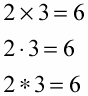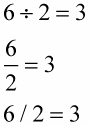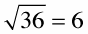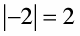##### Algebra I For DummiesThe basics of algebra involve symbols. Algebra uses symbols for quantities, operations, relations, or grouping. The symbols are shorthand and are much more efficient than writing out the words or meanings. But you need to know what the symbols represent, and the following list shares some of that info.

• Addition (+): This symbol means add or find the sum, more than, or increased by; the result of addition is the sum.

1 + 2 = 3

• Subtraction (–): This symbol means subtract, minus, or decreased by or less; the result is the difference.

3 – 2 = 1

• Multiplication (×, ∙, *): These symbols all mean multiply or times. The values being multiplied together are the multipliers or factors (in this example, 2 and 3), and the result is the product (in this example, 6). You'll see the dot (∙) more often than the times symbol (×) because the dot is easier to write and the times symbol can be confused with the variable x.• Division (÷, −, /): The division, fraction line, and slash symbols all mean divide. The number to the left of the ÷ or / sign or the number on top of the fraction is the dividend (in this example, 6). The number to the right of the ÷ or / sign or the number on the bottom of the fraction is the divisor (in this example, 2). The result is the quotient (in this example, 3).• Radical (√): This symbol means to take the square root of something — to find the number which, multiplied by itself, gives you the number under the sign. Here's an example:• Absolute Value (| |): This symbol means to find the absolute value of the number between the two vertical lines, which is the number itself or its distance from zero on the number line. Absolute values are always positive. Here's an example:• Ellipsis (...): This symbol means et cetera, and so on, or in the same pattern. You use an ellipsis in algebra when you have a long list of numbers and don’t want to have to write all of them.

• Pi (Π): The Greek letter pi refers to the irrational number, 3.14159…. Pi represents the relationship between the diameter and circumference of a circle.